Next: Light Nuclei Up: FEW-NUCLEON SYSTEMS Previous: The Deuteron, and more

## Effective Field Theory

As we have discussed, the Argonne v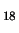interaction uses the OPE potential at large distances, and the phenomenological interaction at intermediate and small distances. One can also follow the standard ideology of the quantum-field theory, and model the second piece by the exchange effects for heavier mesons. Larger meson masses mean shorter distances of the interaction, so we can understand why adding more mesons, and using the corresponding Yukawa interactions, we can parametrize the NN force equally well.

Although this way of proceeding works very well in practice, it creates two conceptual problems. First, one has to include the scalar-isoscalar meson called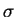, which has the quantum numbers of a pair of pions. It fulfills the role of an exchange of the a pair of pions, however, such a meson neither exist in Nature as a free particle, nor its mass, that has to be used in the corresponding Yukawa term, is close to the doubled pion mass. The exchange of such a virtual particle simply corresponds to higher-order effects in the exchange of pions, which is a perfectly legitimate procedure, but it departs from the idea that real, physical particles mediate the NN interaction.

Second, two other heavy mesons have to be included, namely, the vector isovector meson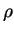and the vector isoscalar meson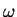. They are physical particles, with the rest masses of about 800MeV, and the corresponding ranges of the Yukawa potentials are very small, of the order of 0.25fm. These small ranges allow to model the NN interaction at very short distances, but at these distances nucleons really start to touch and overlap. Therefore, it is rather unphysical to think that nucleons can still interact as unchanged objects, by exchanging physical particles. Within the image of the strong color-polarization taking place at such a small distances, one would rather think that the internal quark-gluon structure of nucleons becomes strongly affected, which creates strong repulsion effects, predominantly through the Pauli blocking of overlapping quark states.

At present, we are probably not at all able to tell what happens with the nucleons when they are put so near to one another. However, we do not really need such a complete knowledge when describing low-energy NN scattering and structure of nuclei. All what we need is some kind of parameterization of the short-range, high-energy effects when we look at their influence on the long-range, low-energy observables. Such separation of scales is at the heart of the effective field theory (EFT).

One can apply similar ideas to almost all physical systems, where our knowledge of the detailed structure is neither possible nor useful. The simplest example is the effect of the electromagnetic charge and current distributions inside a small object, when we shine at it an electromagnetic wave of a much longer length (the long-wave-length limit). It is well known that all what we then need, are a few numbers - low-multiplicity electric and magnetic moments. Of course, the best would be to be able to calculate these moments from the exact charge and current distributions, but once we know these numbers, we know everything. On the other hand, if the internal structure is not known, we can fit these numbers to the measured long-wave scattering, and thus obtain the complete information needed to describe such a scattering process.

Examples of other such situations are plenty in physics. Interested students are invited to go through very good introductory lecture notes by Lepage Lep97, where nice instructive examples are presented within the framework of ordinary quantum mechanics. In particular, it is shown how a short-range perturbation of the ordinary Coulomb potential influences the hydrogen atomic wave functions, and how such a perturbation (no matter its physical origin) can be parametrized by a zero-range, delta-like potential.

Here we only discuss two applications of the EFT, which pertain to the pion and nucleon systems. First, let us consider the pion-pion Lagrangian density (38). When we use the methods of the quantum field theory to derive the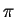-scattering amplitude, it turns out that details of the experimental results are not well reproduced. This suggests that even during a low-energy scattering process of composite particles, the internal, short-range, high-energy structure does become visible. The question is whether one can modify the Lagrangian density in such a way that the internal quark-gluon degrees of freedom do not explicitly appear, and yet their influence on the-scattering amplitude is taken into account. The EFT prescription suggests that one should add to the Lagrangian higher-order terms that depend on the pion field and conserve all symmetries of the theory (Lorentz and chiral invariance in this case). We than obtain the effective Lagrangian density,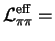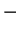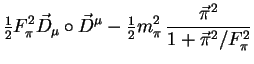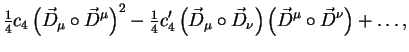(54)

built from the covariant derivatives of the pion field (39), which ensures the chiral invariance, and with all the Lorentz indices summed up in pairs, which ensures the Lorentz invariance. Up to these rules, there are two quartic terms possible, and the series could be, in principle, continued to even higher orders. However, by adjusting free parameters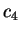and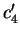we are now able to properly describe the experimental-scattering data. Note that the quartic terms in the local Lagrangian density can be interpreted as zero-range contact (point-like) interactions. In Eq. (54) we have also included the pion mass term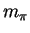, which explicitly (but weakly) breaks the chiral invariance.

The second example concerns the nucleon-pion Lagrangian density (44) that can be transformed into an effective Lagrangian by adding terms which are quartic in the nucleon fields,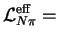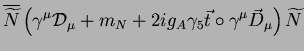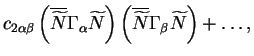(55)

where we have combined two terms of Eq. (44) into the covariant derivative of the nucleon field,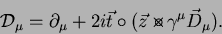(56)

Symbols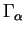and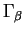denote projection operators on the spin-isospin channels, and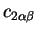are the adjustable free parameters. Again, this Lagrangian contains the effects of the pion Yukawa potential, but apart from that, all other short-range effects are modelled by the point-like contact interactions. This Lagrangian properly describes all NN scattering lengths, not only in the high-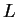partial waves where the OPE potential is enough, but also in the low-partial waves.

Recently, ideas of the EFT for the NN scattering were followed further, by also adding to Lagrangian density (56) terms which contain six nucleon fields, and calculating the full energy dependence of phase shifts and mixing parameters in all partial waves Ent02. The resulting effective Lagrangian density has many adjustable parameters, but the number of these parameters is comparable to that used in the parameterization of Lagrangian by heavy mesons. Also the description of the NN scattering data is of a comparable quality, i.e., very good. This shows that the ideas of the EFT really work; namely, it is not important which physical mechanism is used to model the short-range effects - a purely phenomenological mechanism is equally good. Our knowledge of these short-range effects can be summarized in a form of a certain number of constants that have the meaning of the multipole moments discussed above. Of course, it would be fantastically interesting to calculate these constants from the basic theory (QCD), but the description of low-energy nuclear phenomena requires only that these constants be known, while the whole complication of the vacuum, pion, and nucleon states does not enter the game.Next: Light Nuclei Up: FEW-NUCLEON SYSTEMS Previous: The Deuteron, and more
Jacek Dobaczewski 2003-01-27